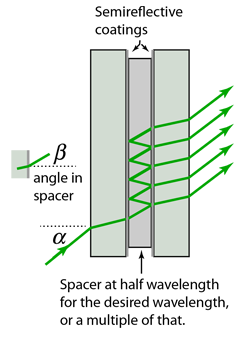# Interference FiltersIf a thin transparent spacer is placed between two semireflective coatings, multiple reflections and interference can be used to select a narrow frequency band, producing an interference filter. If the spacer is a half wavelength for the desired wavelength, then other wavelengths will be attenuated by destructive interference. Commercial filters are available with a half-power width of about an angstrom. If the back layer is totally reflective, then the arrangement is called a dichroic mirror, reflecting only the selected wavelength. These devices are designed for normal incidence, and shift in wavelength to shorter wavelengths if tilted.

 Calculation
 Example of image taken through an interference filter
Index

Interference concepts

Modern Optics Concepts

 HyperPhysics***** Light and Vision R Nave
Go Back

# Interference FiltersInterference filter discussion

An interference filter to pass

 λ = nm

can be produced with a spacer of index

 n =

with thickness calculated from the interference condition:= nm for normal incidence (β = α = 0).
 However, if the angle of incidence is α = °,

the passed wavelength is given by= nm

Index

Interference concepts

 HyperPhysics***** Light and Vision R Nave
Go Back